login  home  contents  what's new  discussion  bug reports help  links  subscribe  changes  refresh  edit

## Translation from Somos notation to eta-function notation and back

Related to http://www.risc.jku.at/people/hemmecke/eta/ and EtaRelations8 .

We demonstrate below how :

    t8_12_24 = +1*u1^8*u4^4 +8*q*u1^4*u2^2*u4^2*u8^4 -1*u2^12


can be translated into :

    8*E1^4*E2^2*E4^2*E8^4+E1^8*E4^4-E2^12


fricas
(1) -> <spad>
-------------------------------------------------------------------
---
--- FriCAS QEta
--- Copyright (C) 2015-2017  Ralf Hemmecke <ralf@hemmecke.org>
---
-------------------------------------------------------------------
-- This program is free software: you can redistribute it and/or modify
-- the Free Software Foundation, either version 3 of the License, or
-- (at your option) any later version.
--
-- This program is distributed in the hope that it will be useful,
-- but WITHOUT ANY WARRANTY; without even the implied warranty of
-- MERCHANTABILITY or FITNESS FOR A PARTICULAR PURPOSE.  See the
-- GNU General Public License for more details.
--
-- You should have received a copy of the GNU General Public License
-- along with this program.  If not, see <http://www.gnu.org/licenses/>.
-------------------------------------------------------------------
OF==>OutputForm
dbgPrint(x,y) ==> print([x::OF, y::OF]$List(OF)::OF) rep x ==> (x@%) pretend Rep per x ==> (x@Rep) pretend % P ==> PositiveInteger N ==> NonNegativeInteger Z ==> Integer Q ==> Fraction Z UP ==> SparseUnivariatePolynomial C SQ ==> SquareMatrix(n, Q) DQ ==> DirectProduct(n, Q) VZ ==> Vector Z VQ ==> Vector Q LSym ==> List Symbol  fricas )abbrev package POLYEVAL PolynomialEvaluation PolynomialEvaluation(_ C: Ring, _ S: with (_ _+: (%, %) -> %; _ _*: (%, %) -> %; _ _^: (%, NonNegativeInteger) -> %)_ ): with eval: (Polynomial C, C -> S, LSym, List S) -> S == add eval(p: Polynomial C, embed: C -> S, vars: LSym, vals: List S): S == E ==> IndexedExponents Symbol PE ==> PolynomialCategoryLifting(E, Symbol, C, Polynomial C, S) map((s:Symbol):S +-> vals.position(s, vars), embed, p)$PE

fricas
)abbrev package STOETA SomosToEta
SomosToEta: Exports == Implementation where
Pol ==> Polynomial Z
LPol ==> List Pol
Exports ==> with
toEta: (N, Pol) -> Pol
++ toEta(p) expresses p (given as a polynomial in variables ui and q
++ where the ui correspond to the Euler function
++ https://en.wikipedia.org/wiki/Euler_function) into an expression
++ in variables Ei (corresponding to eta(i*tau)).
fromEta: (N, Pol) -> Pol
++ fromEta(p) expresses a polynomial p in variables Ei
++ (corresponding to eta(i*tau)) in terms of variables q and ui
++ where the ui correspond to the Euler function
++ https://en.wikipedia.org/wiki/Euler_function).
indexedSymbols(s: String, n: N): List Symbol ==
[concat(s, convert(i)@String)::Symbol for i in 1..n]
indexedSymbols(s: String, l: List Z): List Symbol ==
[concat(s, convert(i)@String)::Symbol for i in l]
-- toEta(level, p) works by substituting E_d*t^d for u_d and
-- t^(-24) for q. This gives a polynomial in t and the E
-- variables. If everything is OK, the result should be a
-- polynomial in the E variables times a power of t. We only
-- return the factor that does not involve t.
toEta(level: N, p: Pol): Pol ==
import from QAuxiliaryTools
FPol ==> Fraction Pol
divs: List Z := divisors(level)$IntegerNumberTheoryFunctions usyms: LSym := indexedSymbols("u", divs) esyms: LSym := indexedSymbols("E", divs) fc(c: Z): FPol == c::FPol syms: LSym := cons("q"::Symbol, usyms) evals: List FPol := [e::Pol::FPol for e in esyms] symt: Symbol := "t"::Symbol t: Pol := symt::Pol tt := t::FPol -- 1/q = t^24 vals: List FPol := [e*tt^d for e in evals for d in divs] vals := cons(inv(tt^24), vals) z: FPol := eval(p, fc, syms, vals)$PolynomialEvaluation(Z, FPol)
if not one? denom z then error "toEta: denominator is not 1"
x: Pol := numer z
z: Union(Pol, "failed") := x exquo t
k: N := 0
while z case Pol repeat
k := k + 1
x := z :: Pol
z := x exquo t
dbgPrint("toEta: power of t", k)
if member?(symt, variables x) then
dbgPrint("debug message: toEta$CheckSomos contains t", x) return x fromEta(level: N, p: Pol): Pol == import from QAuxiliaryTools divs: List Z := divisors(level)$IntegerNumberTheoryFunctions
usyms: LSym := indexedSymbols("u", divs)
esyms: LSym := indexedSymbols("E", divs)
fc(c: Z): Pol == c::Pol
uvals: LPol := [u::Pol for u in usyms]
symt: Symbol := "t"::Symbol
t: Pol := symt::Pol
vals: LPol := [u*t^(qcoerce(d)@N) for u in uvals for d in divs]
x: Pol := eval(p, fc, esyms, vals)\$PolynomialEvaluation(Z, Pol)
return x</spad>
fricas
Compiling FriCAS source code from file
using old system compiler.
POLYEVAL abbreviates package PolynomialEvaluation
------------------------------------------------------------------------
initializing NRLIB POLYEVAL for PolynomialEvaluation
compiling into NRLIB POLYEVAL
compiling exported eval : (Polynomial C,C -> S,List Symbol,List S) -> S
processing macro definition E ==> IndexedExponents Symbol
processing macro definition PE ==> PolynomialCategoryLifting(IndexedExponents Symbol,Symbol,C,Polynomial C,S)
Time: 0.03 SEC.
(time taken in buildFunctor:  0)
;;;     ***       |PolynomialEvaluation| REDEFINED
;;;     ***       |PolynomialEvaluation| REDEFINED
Time: 0 SEC.
Cumulative Statistics for Constructor PolynomialEvaluation
Time: 0.03 seconds
finalizing NRLIB POLYEVAL
Processing PolynomialEvaluation for Browser database:
--->-->PolynomialEvaluation(constructor): Not documented!!!!
--->-->PolynomialEvaluation((+ (% % %))): Not documented!!!!
--->-->PolynomialEvaluation((* (% % %))): Not documented!!!!
--->-->PolynomialEvaluation((^ (% % (NonNegativeInteger)))): Not documented!!!!
--->-->PolynomialEvaluation((eval (S (Polynomial C) (Mapping S C) (List (Symbol)) (List S)))): Not documented!!!!
--->-->PolynomialEvaluation(): Missing Description
; compiling file "/var/aw/var/LatexWiki/POLYEVAL.NRLIB/POLYEVAL.lsp" (written 12 OCT 2023 12:06:13 PM):
; wrote /var/aw/var/LatexWiki/POLYEVAL.NRLIB/POLYEVAL.fasl
; compilation finished in 0:00:00.012
------------------------------------------------------------------------
PolynomialEvaluation is now explicitly exposed in frame initial
PolynomialEvaluation will be automatically loaded when needed from
/var/aw/var/LatexWiki/POLYEVAL.NRLIB/POLYEVAL
STOETA abbreviates package SomosToEta
------------------------------------------------------------------------
initializing NRLIB STOETA for SomosToEta
compiling into NRLIB STOETA
compiling local indexedSymbols : (String,NonNegativeInteger) -> List Symbol
Time: 0.01 SEC.
compiling local indexedSymbols : (String,List Integer) -> List Symbol
Time: 0.00 SEC.
compiling exported toEta : (NonNegativeInteger,Polynomial Integer) -> Polynomial Integer
processing macro definition FPol ==> Fraction Polynomial Integer
Local variable z type redefined: (Union (Polynomial (Integer)) failed) to (Fraction (Polynomial (Integer)))
Time: 0.04 SEC.
compiling exported fromEta : (NonNegativeInteger,Polynomial Integer) -> Polynomial Integer
Time: 0.00 SEC.
(time taken in buildFunctor:  0)
;;;     ***       |SomosToEta| REDEFINED
;;;     ***       |SomosToEta| REDEFINED
Time: 0.00 SEC.
Cumulative Statistics for Constructor SomosToEta
Time: 0.06 seconds
finalizing NRLIB STOETA
Processing SomosToEta for Browser database:
--->-->SomosToEta(constructor): Not documented!!!!
--------(toEta ((Polynomial (Integer)) (NonNegativeInteger) (Polynomial (Integer))))---------
--------(fromEta ((Polynomial (Integer)) (NonNegativeInteger) (Polynomial (Integer))))---------
--->-->SomosToEta((fromEta ((Polynomial (Integer)) (NonNegativeInteger) (Polynomial (Integer))))): Missing left pren
--->-->SomosToEta(): Missing Description
; compiling file "/var/aw/var/LatexWiki/STOETA.NRLIB/STOETA.lsp" (written 12 OCT 2023 12:06:13 PM):
; wrote /var/aw/var/LatexWiki/STOETA.NRLIB/STOETA.fasl
; compilation finished in 0:00:00.024
------------------------------------------------------------------------
SomosToEta is now explicitly exposed in frame initial
SomosToEta will be automatically loaded when needed from
/var/aw/var/LatexWiki/STOETA.NRLIB/STOETA

fricas
t8_12_24 := u1^8*u4^4 +8*q*u1^4*u2^2*u4^2*u8^4 -1*u2^12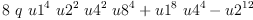(1)
Type: Polynomial(Integer)
fricas
T8_12_24 := toEta(8, t8_12_24)
["toEta: power of t", 24]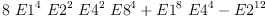(2)
Type: Polynomial(Integer)
fricas
A8_12_24 := fromEta(8, T8_12_24)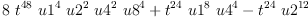(3)
Type: Polynomial(Integer)

The variable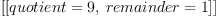is such that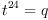.

fricas
B8_12_24 := eval(A8_12_24, t=q^(1/24))::Polynomial(Integer)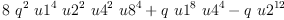(4)
Type: Polynomial(Integer)
fricas
C8_12_24 := factorList factor B8_12_24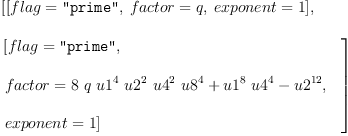(5)
Type: List(Record(flag: Union("nil","sqfr","irred","prime"),factor: Polynomial(Integer),exponent: NonNegativeInteger?))
fricas
q* t8_12_24 - B8_12_24(6)
Type: Polynomial(Integer)

 Subject:   Be Bold !! ( 14 subscribers )